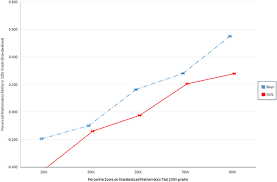# How to Calculate and Solve for TEX | Denier | Polymer & TextileThe image above represents TEX | Denier.

To compute for TEX | Denier, one essential parameter is needed and this parameter is denier (D).

The formula for calculating the TEX | Denier:

TEX = Denier / 9

Where;

TEX = TEX
D = Denier

Let’s solve an example;
Given that denier is 12. Find the TEX | Denier?

This implies that;

D = Denier = 12

TEX = Denier / 9
TEX = 12 / 9
TEX = 1.33

Therefore, the TEX is 1.33.

Calculating the Denier when the TEX is Given.

D = TEX x 9

Where;

TEX = TEX
D = Denier

Let’s solve an example;
Find the denier when the TEX is 27.

This implies that;

D = TEX x 9
D = 27 x 9
D = 243

Therefore, the denier is 243.

Nickzom Calculator – The Calculator Encyclopedia is capable of calculating the TEX | Denier.

To get the answer and workings of the TEX | Denier using the Nickzom Calculator – The Calculator Encyclopedia. First, you need to obtain the app.

You can get this app via any of these means:

To get access to the professional version via web, you need to register and subscribe for NGN 1,500 per annum to have utter access to all functionalities.
You can also try the demo version via https://www.nickzom.org/calculator

Apple (Paid) – https://itunes.apple.com/us/app/nickzom-calculator/id1331162702?mt=8
Once, you have obtained the calculator encyclopedia app, proceed to the Calculator Map, then click on Polymer & Textile under Engineering.Now, Click on TEX | Denier under Polymer & TextileThe screenshot below displays the page or activity to enter your value, to get the answer for the TEX | Denier according to the respective parameter which is the denier (D).Now, enter the value appropriately and accordingly for the parameter as required by the denier (D) is 12.Finally, Click on CalculateAs you can see from the screenshot above, Nickzom Calculator– The Calculator Encyclopedia solves for the TEX | Denier and presents the formula, workings and steps too.